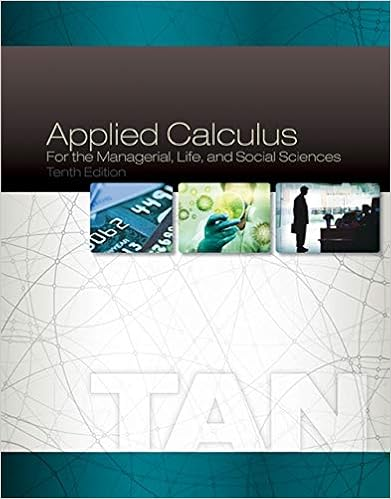# marks Consider the function f x = 5 ln x x 2 a State...

• Lab Report
• 2
• 83% (6) 5 out of 6 people found this document helpful

This preview shows page 1 - 2 out of 2 pages.

##### We have textbook solutions for you!
The document you are viewing contains questions related to this textbook.The document you are viewing contains questions related to this textbook.
Chapter 5 / Exercise 58
Applied Calculus for the Managerial, Life, and Social Sciences
TanExpert Verified
Would you like this assignment Graded? YES or NO MA103 Lab Report 7 - Curve Sketching; Optimization Name: Student Number: Spring 2017 1. [13 marks ] Consider the function f ( x ) = 5 ln x x 2 . (a) State the domain of f using interval notation.
(b) Find all (i) horizontal and (ii) vertical asymptotes of f .
(c) Use Maple to determine f 0 ( x ) and f 00 ( x ) in simplified forms. (First, define the function: f:=(x)- > ???????
(d) Use Maple to determine the critical values of both f ( x ) and f 0 ( x ). ( solve( equation ,x); )
(e) Determine the interval(s) on which f is increasing and on which f is decreasing. State any relative maxi- mum/minimum values. Justify your answers and leave results as exact values.
##### We have textbook solutions for you!
The document you are viewing contains questions related to this textbook.The document you are viewing contains questions related to this textbook.
Chapter 5 / Exercise 58
Applied Calculus for the Managerial, Life, and Social Sciences
TanExpert Verified
•••